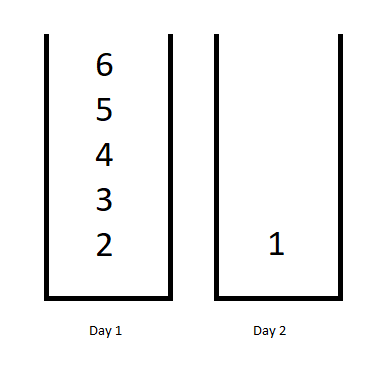1335. Minimum Difficulty of a Job Schedule

You want to schedule a list of jobs in `d` days. Jobs are dependent (i.e To work on the `i-th` job, you have to finish all the jobs `j` where `0 <= j < i`).

You have to finish at least one task every day. The difficulty of a job schedule is the sum of difficulties of each day of the `d` days. The difficulty of a day is the maximum difficulty of a job done in that day.

Given an array of integers `jobDifficulty` and an integer `d`. The difficulty of the `i-th` job is `jobDifficulty[i]`.

Return the minimum difficulty of a job schedule. If you cannot find a schedule for the jobs return -1.

Example 1:```Input: jobDifficulty = [6,5,4,3,2,1], d = 2
Output: 7
Explanation: First day you can finish the first 5 jobs, total difficulty = 6.
Second day you can finish the last job, total difficulty = 1.
The difficulty of the schedule = 6 + 1 = 7
```

Example 2:

```Input: jobDifficulty = [9,9,9], d = 4
Output: -1
Explanation: If you finish a job per day you will still have a free day. you cannot find a schedule for the given jobs.
```

Example 3:

```Input: jobDifficulty = [1,1,1], d = 3
Output: 3
Explanation: The schedule is one job per day. total difficulty will be 3.
```

Example 4:

```Input: jobDifficulty = [7,1,7,1,7,1], d = 3
Output: 15
```

Example 5:

```Input: jobDifficulty = [11,111,22,222,33,333,44,444], d = 6
Output: 843
```

Constraints:

• `1 <= jobDifficulty.length <= 300`
• `0 <= jobDifficulty[i] <= 1000`
• `1 <= d <= 10`

1335. Minimum Difficulty of a Job Schedule
``````struct Solution;

use std::collections::HashMap;

impl Solution {
fn min_difficulty(job_difficulty: Vec<i32>, d: i32) -> i32 {
let n = job_difficulty.len();
let d = d as usize;
if d > n {
return -1;
}
let mut memo: HashMap<(usize, usize), i32> = HashMap::new();
Self::dp(0, d, &mut memo, &job_difficulty, n)
}

fn dp(
start: usize,
d: usize,
memo: &mut HashMap<(usize, usize), i32>,
jobs: &[i32],
n: usize,
) -> i32 {
if let Some(&res) = memo.get(&(start, d)) {
return res;
}
let res = if d == 1 {
*jobs[start..n].iter().max().unwrap()
} else {
if start + d == n {
jobs[start..start + d].iter().sum()
} else {
let mut min = std::i32::MAX;
let mut max = 0;
for i in start..=(n - d) {
max = max.max(jobs[i]);
min = min.min(max + Self::dp(i + 1, d - 1, memo, jobs, n));
}
min
}
};
memo.insert((start, d), res);
res
}
}

#[test]
fn test() {
let job_difficulty = vec![6, 5, 4, 3, 2, 1];
let d = 2;
let res = 7;
assert_eq!(Solution::min_difficulty(job_difficulty, d), res);
let job_difficulty = vec![9, 9, 9];
let d = 4;
let res = -1;
assert_eq!(Solution::min_difficulty(job_difficulty, d), res);
let job_difficulty = vec![1, 1, 1];
let d = 3;
let res = 3;
assert_eq!(Solution::min_difficulty(job_difficulty, d), res);
let job_difficulty = vec![7, 1, 7, 1, 7, 1];
let d = 3;
let res = 15;
assert_eq!(Solution::min_difficulty(job_difficulty, d), res);
let job_difficulty = vec![11, 111, 22, 222, 33, 333, 44, 444];
let d = 6;
let res = 843;
assert_eq!(Solution::min_difficulty(job_difficulty, d), res);
}
``````